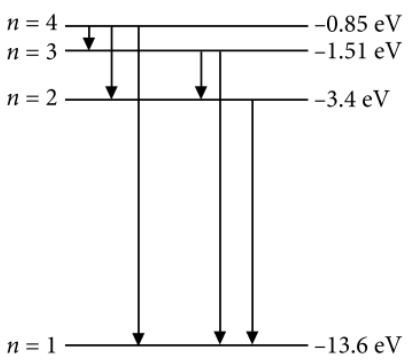Hydrogen -like ion
Question

# Hydrogen atom in ground state is excited by a monochromatic radiation of $\lambda$= 975 $\stackrel{ο}{A}$. Number of spectral lines in the resulting spectrum emitted will be

Moderate
Solution

## $=\frac{6.63×{10}^{-34}×3×{10}^{8}}{975×{10}^{-10}×1.6×{10}^{-19}}\mathrm{eV}=12.75\mathrm{eV}$After absorbing a photon of energy 1275 eV, the electron will reach to third excited state of energy-0.85 eV, since energy difference corresponding to n =1 and n = 4 is 12.75 eV. $\therefore$ Number of spectral lines emitted$=\frac{\left(n\right)\left(n-1\right)}{2}=\frac{\left(4\right)\left(4-1\right)}{2}=6$Get Instant Solutions